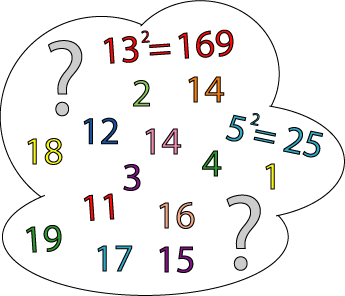#### You may also like### Prompt Cards

These two group activities use mathematical reasoning - one is numerical, one geometric.### Consecutive Numbers

An investigation involving adding and subtracting sets of consecutive numbers. Lots to find out, lots to explore.### Exploring Wild & Wonderful Number Patterns

EWWNP means Exploring Wild and Wonderful Number Patterns Created by Yourself! Investigate what happens if we create number patterns using some simple rules.

# Seven Square Numbers

## Seven Square NumbersAdd the sum of the squares of four numbers between $10$ and $20$ to the sum of the squares of three numbers less than $6$ to make the square of another, larger, number.

### Why do this problem?

This problem is suitable for learners who need a challenge when working with square numbers.

### Key questions

Have you made a list of squares of the relevant numbers?

Have you remembered that $1$ is a square number?

What is the square root of that number?

### Possible extension

Learners who need even more of a challenge could try Cycling squares.

### Possible support

Suggest making a list of squares of the relevant numbers, remembering that $1$ is a square number.